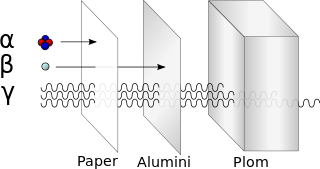Chemistry Level 2Calculate the number of $\alpha$ and $\beta$ particles emitted by the conversion of $^{238}_{92}U\rightarrow^{206}_{82}Pb$

Let the number of $\alpha$ and $\beta$ particles be $x$ and $y$ respectively. Find $x+y$.

×